## Exploring 3x3x3-Like Puzzles (Part I—Orientability)

### Abstract

In this article, we first define 3x3x3-like puzzles (333LPs) and regular 3x3x3-like puzzles (R333LPs), which both generalize the 3x3x3 and the Megaminx. We then explore several R333LPs embedded on different surfaces using Roice Nelson’s MagicTile program. We note how the solving experience changes for the different puzzles depending on their orientability and their size, and in the end, list off R333LPs most similar to the 3x3x3 and the Megaminx.

TL;DR: The second paragraph of the Conclusion section at the end of this article lists out the puzzles that both behave similarly to the 3x3x3 and the Megaminx, and are viewable using MagicTile.

### Regular 3x3x3-Like Puzzles (R333LPs)

We define a 3x3x3-like puzzle (333LP) as a twisty puzzle that is “similar enough” to the 3x3x3 and the Megaminx.

By “similar enough”, we require that:

1. The puzzle has exactly three piece types: edges, corners, and centers.
2. The puzzle is face-turning.
3. There is only one center piece per face.
4. The puzzle can be solved using exactly an edges-first corners-second approach. The puzzle does not have corners with fixed permutations relative to each other.

A regular 333LP, or an R333LP, is then a 333LP that is face-transitive, meaning that all faces on the puzzle have the same number of sides. In other words, R333LPs are an immediate generalization of both the 3x3x3 and the Megaminx.

All R333LPs presented in this article can first be solved by intuitively placing the edges, and then using variations on [[R’, F], D] and/or [[R: U], D] to solve the corners.

### Surfaces and Orientability

We use Roice Nelson’s MagicTile program to try out R333LPs on various surfaces. In this article, we try R333LPs on the sphere, the projective plane, the torus, the Klein bottle, and the hyperbolic plane.

A feature that comes up often when exploring these various surfaces is orientability. We say a surface is non-orientable if a 2D figure can be placed on it at some starting point, and then moved around the surface in such a way that the figure arrives back at the starting point mirror-reversed. Examples of non-orientable surfaces include the Möbius strip and Klein bottle. Orientable surfaces are thus the opposite of non-orientable surfaces. For orientable surfaces, 2D figures placed on some starting point cannot come back to that same point mirror-reversed.

### Tilings and Schläfli Symbols

We use the Schläfli symbol to denote the various tilings explored in this article. The Schläfli symbol $$\{p, q\}$$ represents a tiling with $$q$$ $$p$$-gons per vertex, where a $$p$$-gon is a $$p$$-sided polygon. It turns out that all R333LPs require $$q=3$$ to obtain 3x3x3-like cuts, so we can focus merely on $$\{p, 3\}$$ tilings instead.

### Twisting Single Corners on Non-Orientable Puzzles

To twist a single corner on non-orientable R333LPs, we can first twist two corners. Using a commutator such as [[R’, F]2, D] or [[R, U]2, D], one corner will be twisted clockwise, and the other counterclockwise. Then, we can bring one of the corners to the “opposite” side of the puzzle, which mirrors it due to non-orientability. We can then untwist the two corners with the same commutator, leaving only one of the corners twisted after undoing the setup moves. An example of this state is shown in Figure 1 below.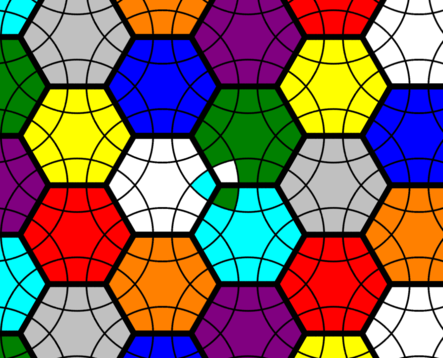Figure 1: A 9-coloured $$\{6,3\}$$ Klein bottle puzzle with a single corner twisted due to non-orientability.

Note that a single corner cannot be twisted on orientable R333LPs since the corner orientation of a single corner is dependent on that of all the other corners, meaning if all but the last corner is solved, then the last corner must be solved too (unless the puzzle is in an illegal/unsolvable permutation).

### Examples of R333LPs

#### The Sphere

The sphere is an orientable surface. We can tile the sphere with $$\{p, 3\}$$ tilings for $$p \in \{3,4,5\}$$. When $$p=3$$ we obtain a tetrahedron, when $$p=4$$ we obtain a cube, and when $$p=5$$ we obtain a dodecahedron. However, we can discard the $$p=3$$ case since the face-turning tetrahedron is Jing’s Pyraminx, which has corners with fixed permutations relative to each other, making it not an R333LP by definition.

Therefore, the spherical R333LPs are exactly the 3x3x3 (Figure 2.a) and the Megaminx (Figure 2.b). However, they appear different in this program due to the use of stereographic projection.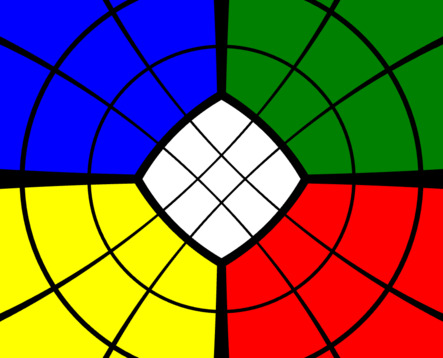Figure 2.a: A 3x3x3 in MagicTile, based off a $$\{4,3\}$$ stereographically projected onto a plane.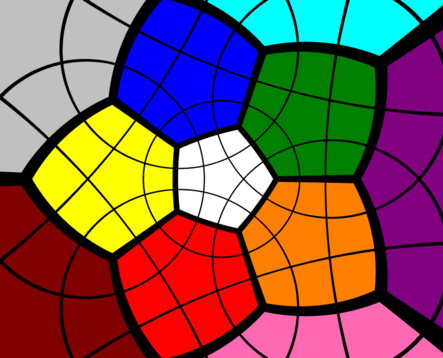Figure 2.b: A Megaminx in MagicTile, based off a $$\{5,3\}$$ stereographically projected onto a plane.

Considering that these R333LPs are the only ones that can be physically produced, we can use these puzzles as a reference point for the other R333LPs below. For the purpose of this article, we claim an R333LP is “not limited in size” if both [[R’, F], D] and [[R: U], D] can be executed from all angles, and only cycle three corners each time. Otherwise, we claim that the R333LP is “limited in size”.

Both the 3x3x3 and the Megaminx are not limited in size by the definition above.

#### The Projective Plane

The projective plane is a non-orientable surface that can be thought of as a sphere where opposite points are the exact same point. For example, whereas we can project a cube and dodecahedron onto a sphere, we can project a hemicube and a hemidodecahedron onto the projective plane. The hemicube is an abstract polyhedron with three faces, but we can represent it as a cube where opposite edges are the same edge, opposite vertices are the same vertex, and opposite faces are the same face. The hemidodecahedron and dodecahedron relate to each other in a similar way.

Then, based on the hemicube and the hemidodecahedron, we can construct the 3x3x3 hemicube (Figure 3.a) and the Hemimegaminx (Figure 3.b). In MagicTile, these puzzles are represented as spherical puzzles where opposite faces are the same face. Thus, if one face is rotated clockwise, the opposite face rotates counterclockwise simultaneously.

The 3x3x3 hemicube is limited in size but trivial, for it can be boiled down into a three-colour slice-turning 3x3x3. Therefore, the corners are always fixed in permutation relative to each other, making this puzzle not an R333LP by definition. (Sure, the 3x3x3 hemicube is like a 3x3x3, but it does not follow the R333LP generalization well.)

Meanwhile, the Hemimegaminx is an R333LP. It can be solved with an edges-first approach, where the corners are afterwards solved using the commutator [[R’, F], D] but not [[R: U], D]; the latter does not work on the Hemimegaminx because it is limited in size. The Hemimegaminx can also be left with one twisted corner at the end of a solve because it is non-orientable.

#### The Torus

On the torus, solving the R333LPs, which take the form of $$\{6,3\}$$ tilings, is fairly straightforward for the most part. Note that we can represent puzzles on the torus by unfolding and stretching the torus into a flat torus.

For the 7-coloured torus (Figure 4.a), after the edges are solved, the corners can be placed only using [[R’, F], D], because the puzzle is limited in size. The 16-coloured torus (Figure 4.b), however, is not limited in size, and can be solved in the same way as the Megaminx.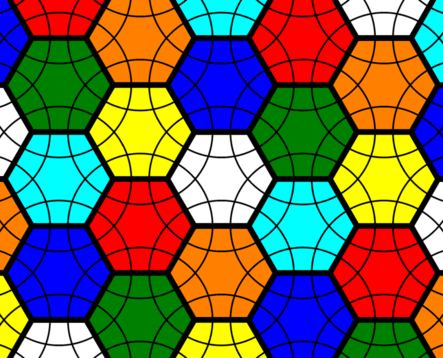Figure 4.a: A 7-coloured $$\{6,3\}$$ flat torus puzzle in MagicTile.Figure 4.b: A 16-coloured $$\{6,3\}$$ flat torus puzzle in MagicTile.

The 6-coloured torus (Figure 4.c), meanwhile, is so limited in size that the only way to really place corners on such a puzzle after the edges are solved is to use careful applications of [[R: U], D].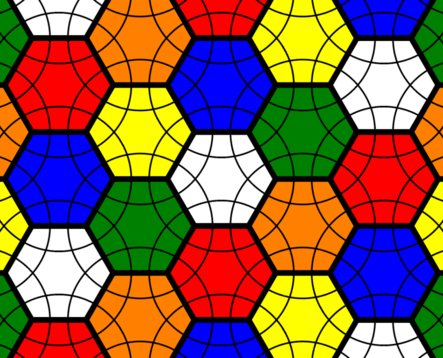Figure 4.c: A 6-coloured $$\{6,3\}$$ flat torus puzzle in MagicTile.

#### The Klein Bottle

The Klein bottle is non-orientable, so once again it is possible to leave only one corner unsolved. We can represent it in a flat plane in a similar way to the flat torus above. On the 9-coloured $$\{6,3\}$$ Klein bottle (Figure 5), both [[R’, F], D] and [[R: U], D] can be used, although the latter cannot be used from all execution angles because the puzzle is limited in size.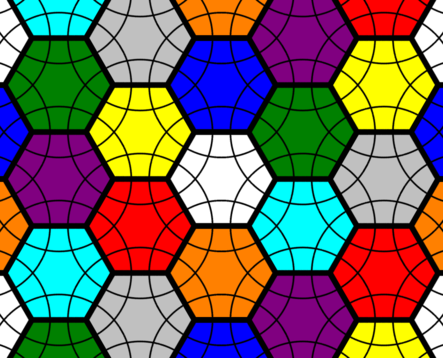Figure 5: A 9-coloured $$\{6,3\}$$ flat Klein bottle puzzle in MagicTile.

#### The Hyperbolic Plane

The hyperbolic plane offers the most R333LPs, merely because its negative curvature allows for any $$\{p,3\}$$ tiling where $$p \ge 7$$. Fortunately, the program offers a way to rotate the puzzle so that the solver is not forced to zoom in to inspect “distant” faces.

We now list a few of the R333LPs that are not limited in size that are available on MagicTile: the 24-coloured $$\{7,3\}$$ (Figure 6.a), the 24-coloured $$\{8,3\}$$, the 18-coloured $$\{10,3\}$$, and the 36-coloured $$\{10,3\}$$ (Figure 6.b).

The 24-coloured $$\{7,3\}$$ has fewer cubies than the Tuttminx, and the 24-coloured $$\{8,3\}$$ has more cubies than the Tuttminx. Meanwhile, the 12-coloured $$\{8,3\}$$ is limited in size in a similar way as the 9-coloured $$\{6,3\}$$ Klein bottle.

The 18-coloured $$\{10,3\}$$ is non-orientable, and the 36-coloured $$\{10,3\}$$ is orientable. The 36-coloured $$\{10,3\}$$ also has the exact same number of cubies (excluding centers) as the truncated icosidodecahedron puzzle. It also has more permutations than the truncated icosidodecahedron puzzle due to its regularity; whereas the former allows for single turns on all of its faces, the latter only admits double turns on all of its faces, and its cubies cannot be re-oriented either due to its non-regular vertex figure.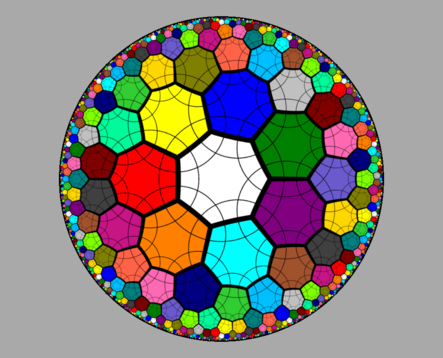Figure 6.a: A 24-coloured $$\{7,3\}$$ hyperbolic plane puzzle in MagicTile.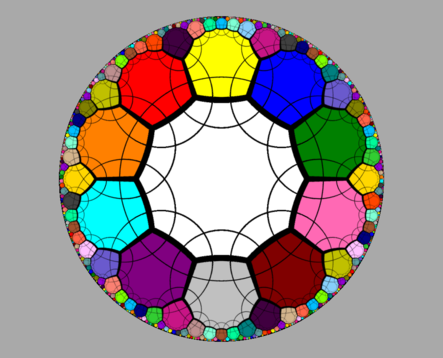Figure 6.b: A 36-coloured $$\{10,3\}$$ hyperbolic plane puzzle in MagicTile.

A feature that immediately stands out is that for large $$p$$, the $$p$$-gons take up more apparent space in the projection used above, which is the Poincaré disk model. In turn, those $$\{p,3\}$$ tilings are more cumbersome to navigate around in MagicTile.

Another feature is that even though the hyperbolic plane admits an infinite number of R333LPs, as $$p$$ increases, moving around the cubies becomes more tedious too. For example, what used to be a U2 on the 3x3x3 or Megaminx could now become a U5 on the 36-coloured $$\{10,3\}$$.

Therefore, the 24-coloured $$\{7,3\}$$ or $$\{8,3\}$$ are probably the most comfortable examples of orientable R333LPs on MagicTile that are not limited in size.

### Conclusions

We looked at regular 3x3x3-like puzzles (R333LPs), which generalize both the 3x3x3 and the Megaminx. R333LPs change in solving properties depending on their orientability and size.

The orientable R333LPs that are not limited in size and are viewable with MagicTile are: the 3x3x3, the Megaminx, the 16-coloured $$\{6,3\}$$ torus, the 24-coloured $$\{7,3\}$$, the 24-coloured $$\{8,3\}$$, and the 36-coloured $$\{10,3\}$$. Since these puzzles are not limited in size, they can all be solved with the exact same edge-first corner-second method, i.e. solving the edges intuitively, and then applying either [[R’, F], D] or [[R: U], D] commutators to solve the corners.

Other R333LPs mentioned were either limited in size, such as the 12-coloured $$\{8,3\}$$; non-orientable, such as the 18-coloured $$\{10,3\}$$; or both, such as the 9-coloured $$\{6,3\}$$ Klein bottle and the Hemimegaminx. Non-orientable R333LPs can have mirrored corners, or be left with a single unsolved corner, both of which increase the difficulty of the solve. R333LPs that are limited in size do not allow execution of the [[R’, F], D] and [[R: U], D] commutators from all angles, which also increases the difficulty of the solve.

### Future Works

In Part II of this series, we plan on using Coxeter-Dynkin diagrams to examine 333LPs based on Archimedean solids and their higher-dimensional counterparts.

Edit (June 3, 2020): Part II is now available.

Go back to Articles